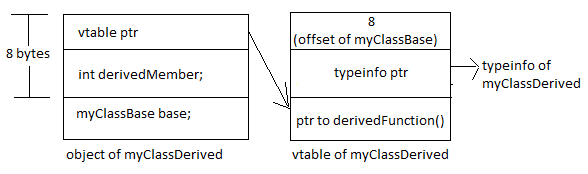# Virtual Base Classes

Let's take this example -
```class myClassBase
{
public:
int baseMember;
};
class myClassDerived : virtual public myClassBase
{
public:
int derivedMember;
int virtual derivedFunction() { return 0; }
};

myClassDerived *o = new myClassDerived;
o->baseMember = 75;
```

The equivalent C code: -
```struct myClassBase
{
int baseMember;
};
struct myClassDerived
{
void *vtable;
int derivedMember;
struct myClassBase base;
};
int myClassDerived_derivedFunction()
{
return 0;
}
struct
{
char *typeName;
}
myClassBase_typeInfo =
{
"myClassBase"
};
struct
{
char *typeName;
void *baseTypeInfo;
}
myClassDerived_typeInfo =
{
"myClassDerived",
&myClassBase_typeInfo
};
struct
{
int myClassBase_offset;
void *typeInfo;
int (*fun1)(struct myClassDerived *);
}
myClassDerived_vtable =
{
8,
&myClassDerived_typeInfo,
myClassDerived_derivedFunction
};
void myClassDerived_ctor(struct myClassDerived *this)
{
this->vtable = (void*)&myClassDerived_vtable + sizeof(int) + sizeof(void*);
}
struct myClassDerived *o = operator_new(sizeof(struct myClassDerived));
myClassDerived_ctor(o);
int offset = *(int*)(o->vtable - (sizeof(int) + sizeof(void*)));
struct myClassBase *base = (void*)o + offset;
base->baseMember = 75;
```

Here is a diagram describing the concept:Points to understand:
• Unlike the non-virtual case, object of derived class does not start with the base class object.
• The base class object offset in derived class object may be anywhere inside the object.
• The offset of the base class object is obtained by a process similar to virtual function resolution.
• The base class object offset is obtained from the vtable. The vtable of the derived class object contains the offset of virtual base class object.
• If there are multiple virtual base classes, the vtable will contain the offset of each base class object in sequence.
• If the base class contains virtual function, then the base class object (which is contained in derived class object) will have a pointer to vtable. And the constructor of derived class will override that vtable pointer.

Question: What is the problem with this code?
```myClassDerived *d = new myClassDerived;
void *ptr = d;
myClassBase *b = (myClassbase*)ptr;
```